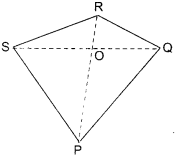NCERT Solutions for Class 6 Maths Chapter 4 Basic Geometrical Ideas Ex 4.5 are part of NCERT Solutions for Class 6 Maths. Here we have given NCERT Solutions for Class 6 Maths Chapter 4 Basic Geometrical Ideas Ex 4.5.

 Board CBSE Textbook NCERT Class Class 6 Subject Maths Chapter Chapter 4 Chapter Name Basic Geometrical Ideas Exercise Ex 4.5 Number of Questions Solved 3 Category NCERT Solutions

## NCERT Solutions for Class 6 Maths Chapter 4 Basic Geometrical Ideas Ex 4.5

Question 1.
Draw a rough sketch of a quadrilateral PQRS. Draw its diagonals. Name them. Is the meeting point of the diagonal in the interior or exterior of the quadrilateral?
Solution :The meeting point O of the diagonals PR and QS of the quadrilateral PQRS is in the interior of the quadrilateral PQRS.

Question 2.
Draw a rough sketch of a quadrilateral KLMN. State :
(a) two pairs of opposite sides.
(b) two pairs of opposite angles.
(c) two pairs of adjacent sides.
(d) two pairs of adjacent angles.
Solution :
(a) $$\bar { KL }$$, $$\bar { NM }$$ and $$\bar { KN }$$, $$\bar { ML }$$
(b) ∠K, ∠M and ∠N, ∠L
(c) $$\bar { KL }$$ , $$\bar { KN }$$ and $$\bar { NM }$$, $$\bar { ML }$$ or $$\bar { KL }$$ , $$\bar { LM }$$ and $$\bar { NM }$$, $$\bar { ML }$$(d) ∠K, ∠L, and ∠M. ∠N or ∠K. ∠L and ∠L, ∠M etc.

Question 3.
Investigate:
Use strips and fasteners to make a triangle and a quadrilateral. Try to push inward at any one vertex of the triangle. Do the same to the quadrilateral. Is the triangle distorted? Is the quadrilateral distorted? Is the triangle rigid? Why is it that structures like electric towers make use of triangular shapes and not quadrilaterals?
Solution :
On pushing inward at any one vertex of the triangle, the triangle is not distorted, However, the quadrilateral is distorted. Hence, a triangle is a rigid figure. This is why structures like electric towers make use of triangular shapes and not quadrilaterals.

We hope the NCERT Solutions for Class 6 Maths Chapter 4 Basic Geometrical Ideas Ex 4.5 help you. If you have any query regarding NCERT Solutions for Class 6 Maths Chapter 4 Basic Geometrical Ideas Ex 4.5, drop a comment below and we will get back to you at the earliest.